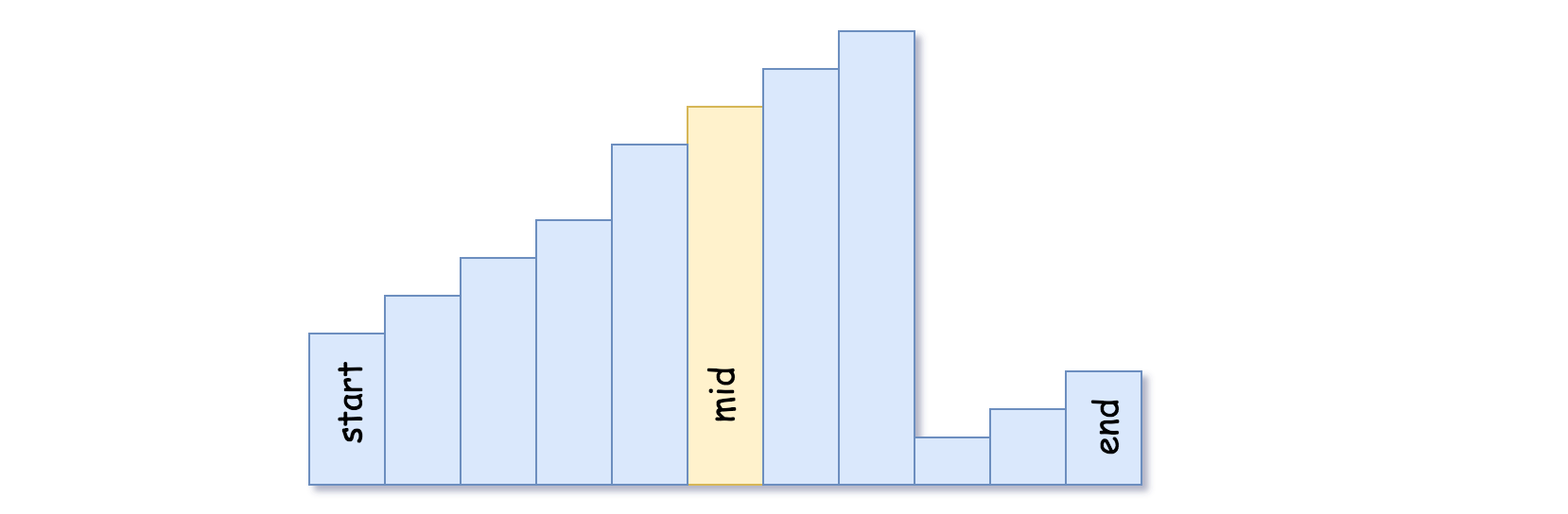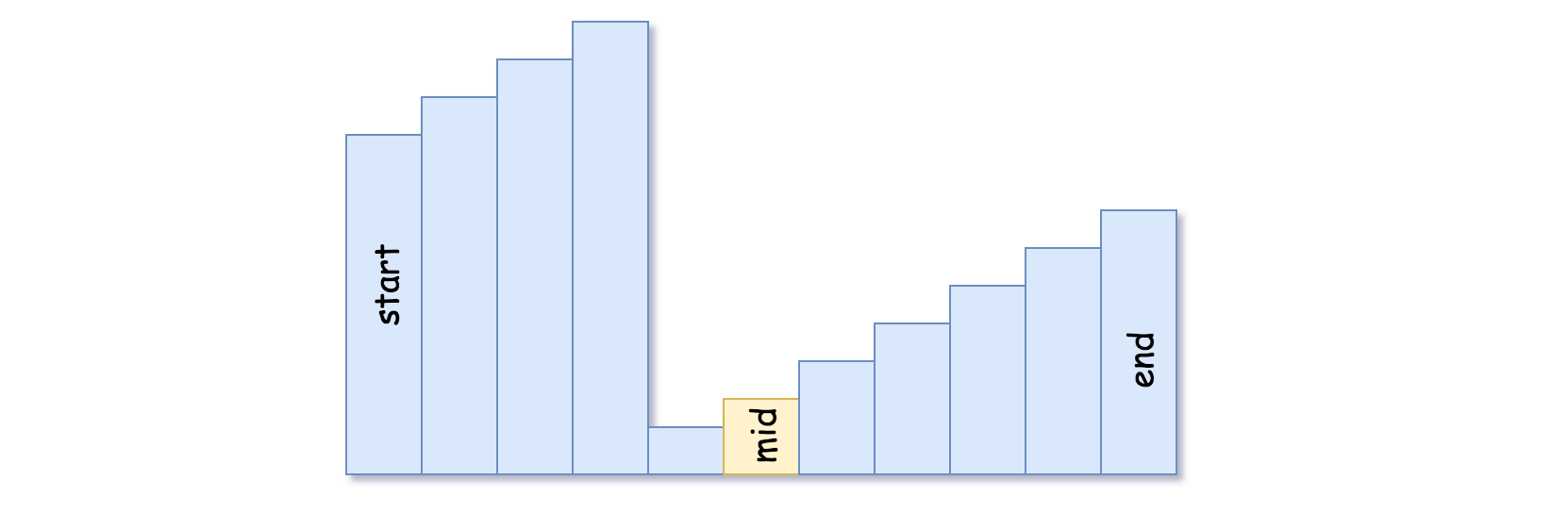### LeetCode• ㊗️
• 大家
• offer
• 多多！

## Problem

There is an integer array nums sorted in ascending order (with distinct values).

Prior to being passed to your function, nums is possibly rotated at an unknown pivot index k (1 <= k < nums.length) such that the resulting array is [nums[k], nums[k+1], …, nums[n-1], nums, nums, …, nums[k-1]] (0-indexed). For example, [0,1,2,4,5,6,7] might be rotated at pivot index 3 and become [4,5,6,7,0,1,2].

Given the array nums after the possible rotation and an integer target, return the index of target if it is in nums, or -1 if it is not in nums.

You must write an algorithm with O(log n) runtime complexity.

Example 1:

Input: nums = [4,5,6,7,0,1,2], target = 0
Output: 4


Example 2:

Input: nums = [4,5,6,7,0,1,2], target = 3
Output: -1


Example 3:

Input: nums = , target = 0
Output: -1


Constraints:

• 1 <= nums.length <= 5000
• $-10^4 <= nums[i] <= 10^4$
• All values of nums are unique.
• nums is an ascending array that is possibly rotated.
• $-10^4 <= target <= 10^4$

## Codeclass Solution {
public int search(int[] nums, int target) {
if(nums == null || nums.length == 0) return -1;

int left = 0;
int right = nums.length - 1;
while(left + 1 < right){
int mid = left + (right - left) / 2;
if(nums[mid] == target) return mid;

if(nums[left] <= nums[mid]){
if(nums[left] <= target && target <= nums[mid]){
right = mid;
} else {
left = mid;
}
} else {
if(nums[mid] <= target && target <= nums[right]){
left = mid;
} else {
right = mid;
}
}
}

if(nums[left] == target){
return left;
} else if (nums[right] == target){
return right;
} else {
return -1;
}
}
}